Author: Oscar Cronquist Article last updated on February 14, 2023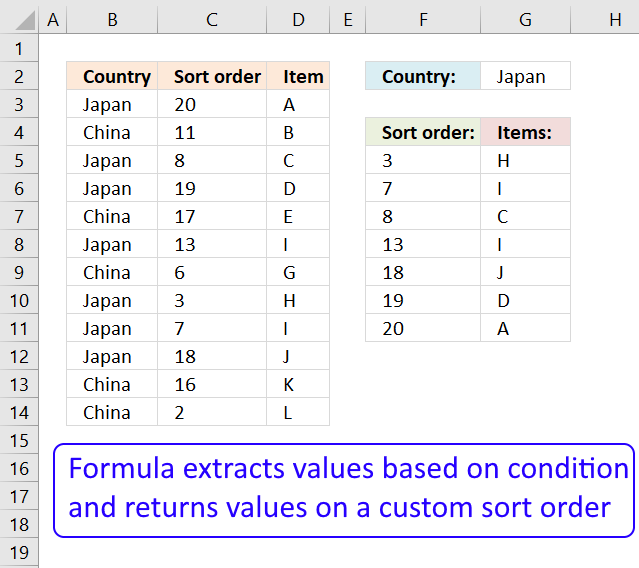This article demonstrates a formula that extracts values based on a condition and sorts the returned values based on values in another column. This article also explains how to apply a filter and sorting to an Excel Table manually.

## 1. Lookup and return multiple sorted values based on corresponding values in another column - Excel 365Excel 365 dynamic array formula:

=LET(x,FILTER(C3:D14, B3:B14=G2),SORTBY(x,INDEX(x,0,1))

### Explaining formula

SORTBY(FILTER(C3:D14, B3:B14=G2),INDEX(FILTER(C3:D14, B3:B14=G2),0,1))

#### Step 1 - Find values equal to condition

The equal sign lets you compare value to value, the result is a boolean value TRUE or FALSE.

B3:B14=G2

becomes

{"Japan"; "China"; "Japan"; "Japan"; "China"; "Japan"; "China"; "Japan"; "Japan"; "Japan"; "China"; "China"}="Japan"

and returns

{TRUE; FALSE; TRUE; TRUE; FALSE; TRUE; FALSE; TRUE; TRUE; TRUE; FALSE; FALSE}

#### Step 2 - Filter values based on logical expression

The FILTER function extracts values/rows based on a condition or criteria.

Function syntax: FILTER(array, include, [if_empty])

FILTER(C3:D14, B3:B14=G2)

becomes

FILTER({20,"A"; 11,"B"; 8,"C"; 19,"D"; 17,"E"; 13,"I"; 6,"G"; 3,"H"; 7,"I"; 18,"J"; 16,"K"; 2,"L"}, {TRUE; FALSE; TRUE; TRUE; FALSE; TRUE; FALSE; TRUE; TRUE; TRUE; FALSE; FALSE})

and returns

{20,"A"; 8,"C"; 19,"D"; 13,"I"; 3,"H"; 7,"I"; 18,"J"}

#### Step 3 - Extract first column in array

The INDEX function returns a value or reference from a cell range or array, you specify which value based on a row and column number.

Function syntax: INDEX(array, [row_num], [column_num])

INDEX(FILTER(C3:D14, B3:B14=G2),0,1)

becomes

INDEX({20,"A"; 8,"C"; 19,"D"; 13,"I"; 3,"H"; 7,"I"; 18,"J"},0,1)

and returns

{20; 8; 19; 13; 3; 7; 18}.

#### Step 4 - Sort array based on first column

The SORTBY function sorts a cell range or array based on values in a corresponding range or array.

Function syntax: SORTBY(array, by_array1, [sort_order1], [by_array2, sort_order2],…)

SORTBY(FILTER(C3:D14, B3:B14=G2),INDEX(FILTER(C3:D14, B3:B14=G2),0,1))

becomes

SORTBY({20,"A"; 8,"C"; 19,"D"; 13,"I"; 3,"H"; 7,"I"; 18,"J"},{20; 8; 19; 13; 3; 7; 18})

and returns

{3, "H"; 7, "I"; 8, "C"; 13, "I"; 18, "J"; 19, "D"; 20, "A"}.

#### Step 5 - Shorten formula

The LET function lets you name intermediate calculation results which can shorten formulas considerably and improve performance.

Function syntax: LET(name1, name_value1, calculation_or_name2, [name_value2, calculation_or_name3...])

SORTBY(FILTER(C3:D14, B3:B14=G2),INDEX(FILTER(C3:D14, B3:B14=G2),0,1))

x - FILTER(C3:D14, B3:B14=G2)

LET(x,FILTER(C3:D14, B3:B14=G2),SORTBY(x,INDEX(x,0,1))

### Get the Excel fileLookup-and-return-multiple-sorted-values-based-on-corresponding-values-in-another-column-Excel-365.xlsx

## 2. Lookup and return multiple sorted values based on corresponding values in another column- previous Excel versionsHi Oscar,
Thanks for creating such a helpful website and I've a question if I would like to return the value with a prefix order would it possible? If not can I just add another column in the data and used it as part of the search criteria?

The array formula in cell G5 looks for the value Japan (cell G2) in column B and returns corresponding values in column D, sorted ascending by the numbers in column C.

Formula in cell G5:

=INDEX(\$D\$3:\$D\$14, MATCH(SMALL(IF(\$G\$2=\$B\$3:\$B\$14, \$C\$3:\$C\$14, ""), ROWS(\$A\$1:A1)), IF(\$G\$2=\$B\$3:\$B\$14, \$C\$3:\$C\$14, ""),0))

Formula in cell F5:

=SMALL(IF(\$G\$2=\$B\$3:\$B\$14, \$C\$3:\$C\$14, ""),ROWS(\$A\$1:A1))

You can change the sort order to descending by replacing the SMALL function with the LARGE function.

#### How to enter an array formula

Excel 365 subscribers do not need to enter the formulas as array formulas.

1. Copy above formula for cell G5 (Ctrl + c).
2. Double press with left mouse button on cell G5.
3. Paste array formula (CTRL + v).
4. Press and hold CTRL + SHIFT simultaneously.
5. Press Enter once.
6. Release all keys.

The formula now has curly brackets before and after the array formula {=array_formula},  if you did the above steps correctly.

#### How to copy array formula to cells below

1. Select cell G5.
2. Copy cell (Ctrl + c).
3. Select cell range G6:G11.
4. Paste (Ctrl + v).

### Explaining array formula in cell G5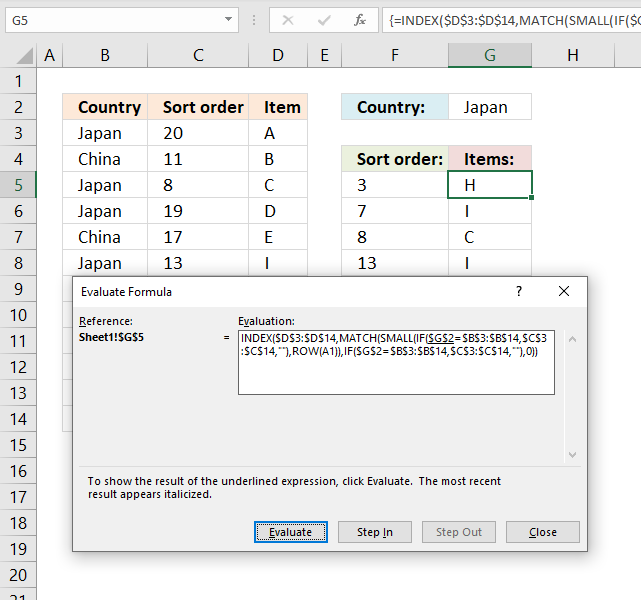The "Evaluate Formula" tool is great for troubleshooting and examining formulas. Select the cell containing the formula you want to debug. Go to tab "Formulas", press with left mouse button on the "Evaluate Formula" button.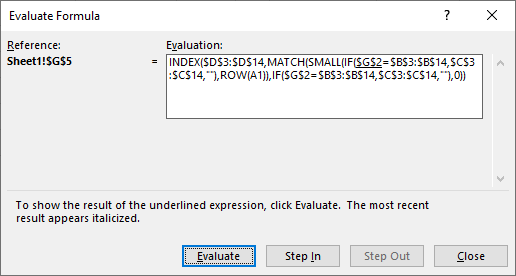A dialog box appears, see image above. Press with left mouse button on the "Evaluate" button move to the next calculation step, underlined expression will be evaluated next when you press with left mouse button on the "Evaluate" button.

Text in italic is the most recent evaluated expression. Keep press with left mouse button oning the "Evaluate" button to see all steps, press with left mouse button on "Close" button to dismiss the dialog box.

#### Step 1 - Filter sort numbers for selected country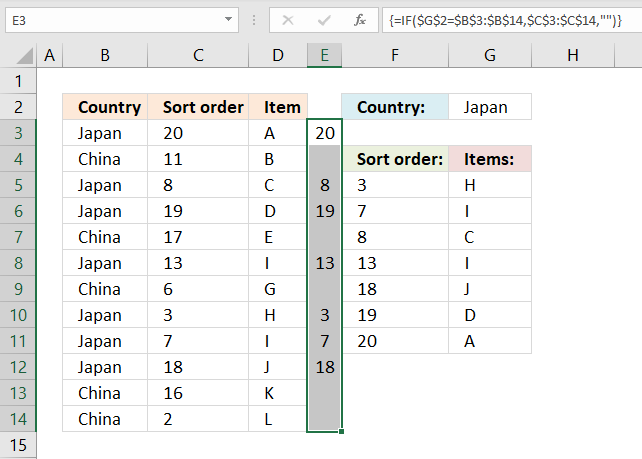The IF function returns one value if the logical expression returns TRUE and another value if FALSE.

IF(logical_test, valie_if_true, value_if_false)

IF(\$G\$2=\$B\$3:\$B\$14,\$C\$3:\$C\$14,"")

becomes

{20; ""; 8; 19; ""; 13; ""; 3; 7; 18; ""; ""}

This array is shown in column E in the image above. Only values from column C are shown based on the condition in cell G2 and the corresponding value in column B.

#### Step 2 - Find k-th smallest value in the array

The SMALL function returns the k-th smallest number from a cell range or an array.

SMALL(array, k)

SMALL(IF(\$G\$2=\$B\$3:\$B\$14, \$C\$3:\$C\$14,""), ROW(A1))

becomes

SMALL({20; ""; 8; 19; ""; 13; ""; 3; 7; 18; ""; ""}, ROW(A1))

becomes

SMALL({20; ""; 8; 19; ""; 13; ""; 3; 7; 18; ""; ""}, 1)

and returns 3.

ROW(A1) changes as we copy the cell to cells below, this makes the array formula dynamic meaning a new value will be returned in each cell.

#### Step 3 - Find the relative position of the k-th smallest value in array

The MATCH function finds the relative position of a value in a column or array.

MATCH(lookup_valuelookup_array[match_type])

MATCH(SMALL(IF(\$G\$2=\$B\$3:\$B\$14, \$C\$3:\$C\$14, ""),ROW(A1)), IF(\$G\$2=\$B\$3:\$B\$14, \$C\$3:\$C\$14, ""), 0)

becomes

MATCH(3, IF(\$G\$2=\$B\$3:\$B\$14, \$C\$3:\$C\$14, ""), 0)

becomes

MATCH(3, {20; ""; 8; 19; ""; 13; ""; 3; 7; 18; ""; ""}, 0)

and returns 8. Number 3 is the eigth value in the array.

#### Step 4 - Return Item

The INDEX function returns a value based on row and column numbers.

INDEX(\$D\$3:\$D\$14, MATCH(SMALL(IF(\$G\$2=\$B\$3:\$B\$14, \$C\$3:\$C\$14, ""),ROW(A1)), IF(\$G\$2=\$B\$3:\$B\$14, \$C\$3:\$C\$14, ""), 0))

becomes

INDEX(\$D\$3:\$D\$14, 8)

becomes

INDEX({"A"; "B"; "C"; "D"; "E"; "I"; "G"; "H"; "I"; "J"; "K"; "L"}, 8)

and returns H in cell G5.

### Get the Excel fileLookup-and-return-multiple-values-sorted-in-a-custom-order.xlsx

## 3. Filter and sort using an Excel Table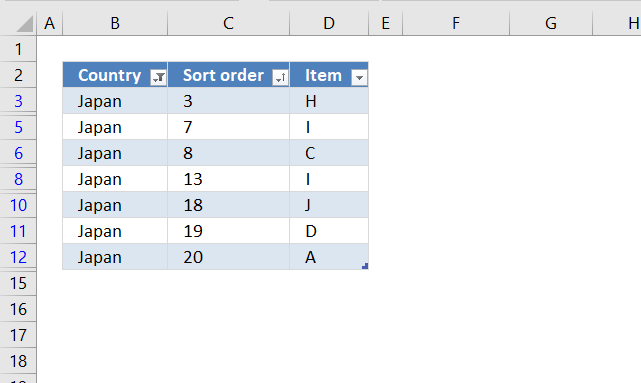The image above shows an Excel Table with a filter applied to column B (Country) and sorting applied to column C (Sort order).

#### How to create an Excel Table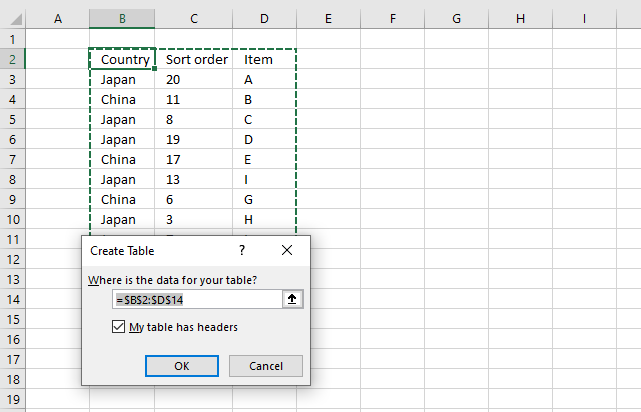1. Press with mouse on any value in the data set.
2. Press CTRL + T to open the "Create Table" dialog box.
3. Press OK button to apply settings and create an Excel Table.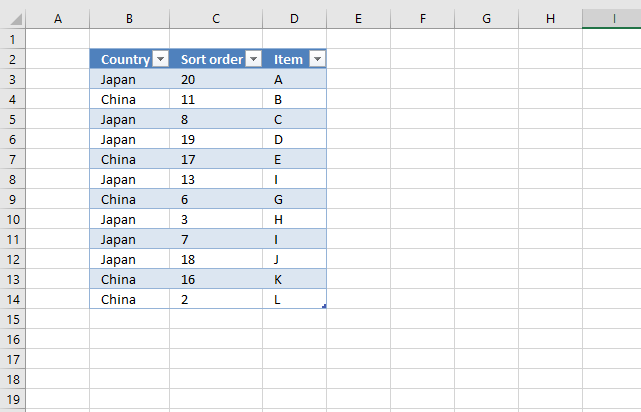#### How to filter an Excel Table based on a condition1. Press with left mouse button on the arrow button next to the column header name you want to filter.
2. Disable all checkboxes except the condition you want to use. I want to filter the table based on item "Japan".
Note, press with left mouse button on the "(Select All)" checkbox to deselect all checkboxes. This saves you time if you have many checkboxes to deselect.3. Press with left mouse button on "OK" button to apply filter conditions.#### How to sort a filtered Excel Table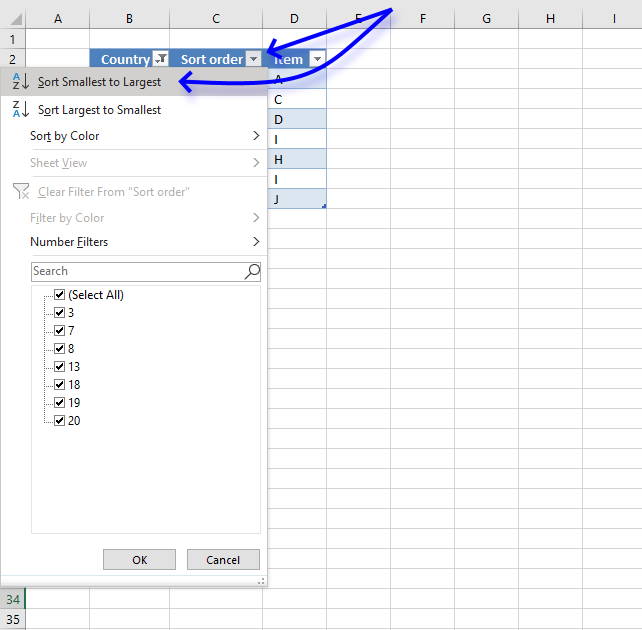1. Press with mouse on the arrow next to the column header name you want to sort by.
2. Press with mouse on "Sort Smallest to Largest" or "Sort Largest to Smallest".
3. Press with left mouse button on OK button to apply sorting.